Students can download 10th Science Chapter 5 Acoustics Questions and Answers, Notes, Samacheer Kalvi 10th Science Guide Pdf helps you to revise the complete Tamilnadu State Board New Syllabus, helps students complete homework assignments and to score high marks in board exams.

## Tamilnadu Samacheer Kalvi 10th Science Solutions Chapter 5 Acoustics

### Samacheer Kalvi 10th Science Acoustics Text Book Back Questions and Answers

Question 1.
When a sound wave travels through air, the air particles:
(a) vibrate along the direction of the wave motion.
(b) vibrate but not in any fixed direction.
(c) vibrate perpendicular to the direction of the wave motion.
(d) do not vibrate.
(a) vibrate along the direction of the wave motion.

Question 2.
The velocity of sound in a gaseous medium is 330 ms-1. If the pressure is increased by 4 times without causing a change in the temperature, the velocity of sound in the gas is _____.
(a) 330 ms-1
(b) 660 ms-1
(c) 156 ms-1
(d) 990 ms-1.
(a) 330 ms-1
Hint: Pressure has no effect on the velocity of sound in the gas. So velocity of sound remains unchanged.

Question 3.
The frequency, which is audible to the human ear is:
(a) 50 kHz
(b) 20 kHz
(c) 15000 kHz
(d) 10000 kHz
(b) 20 kHzQuestion 4.
The velocity of sound in air at a particular temperature is 330 ms-1. What will be its value when the temperature is doubled and the pressure is halved?
(a) 330 ms-1
(b) 165 ms-1
(c) 330 × √2 ms-1
(d) 320/√2 ms-1.
(c) 330 × √2 ms-1
Hint: As there is no effect of change in pressure on the velocity of sound in air, and v ∝ √T therefore, when the temperature is doubled, so the velocity becomes 330√2 m/s.

Question 5.
If a sound wave travels with a frequency of 1.25 × 104 Hz at 344 ms-1, the wavelength will be:
(a) 27.52 m
(b) 275.2 m
(c) 0.02752 m
(d) 2.752 m
(c) 0.02752 m

Question 6.
The sound waves are reflected from an obstacle into the same medium from which they were incident. Which of the following changes?
(a) speed
(b) frequency
(c) wavelength
(d) none of these
(d) none of these

Question 7.
The velocity of sound in the atmosphere of a planet is 500 ms-1. The minimum distance between the sources of sound and the obstacle to hearing the echo should be ______.
(a) 17 m
(b) 20 m
(c) 25 m
(d) 50 m.
(c) 25 m
Hint: The minimum distance of the source of the sound and hear the echo,
$$d=\frac{v t}{2}$$
t = 0.1 s, $$d=\frac{500 \times 0.1}{2}$$ = 25 m.

II. Fill up the blanks:

1. Rapid back and forth motion of a particle about its mean position is called …………
2. If the energy in a longitudinal wave travels from south to north, the particles of the medium would be vibrating in …………
3. A whistle giving out a sound of frequency 450 Hz, approaches a stationary observer at a speed of 33 ms-1. The frequency heard by the observer is (speed of sound = 330 ms-1) ………..
4. A source of sound is travelling with a velocity 40 km/h towards an observer and emits a sound of frequency 2000 Hz. If the velocity of sound is 1220 km/h, then the apparent frequency heard by the observer is …………

1. Wave
2. South to north
3. 500 Hz
4. 2067-3 Hz

III. True or false: (If false give the reason)

1. Sound can travel through solids, gases, liquids and even vacuum.
2. Waves created by Earthquake are Infrasonic.
3. The velocity of sound is independent of temperature.
4. The Velocity of sound is high in gases than liquids.

1. False – Sound cannot travel through vacuum.
2. True
3. False – The velocity of sound depends on temperature.
4. True

IV. Match the following: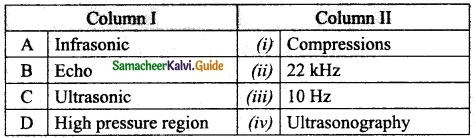A. (iii)
B. (iv)
C. (ii)
D. (i)

V. Assertion and Reason Questions.

Question 1.
Assertion : The change in air pressure affects the speed of sound.
Reason : The speed of sound in a gas is proportional to the square of the pressure.
(a) If both the assertion and the reason are true and the reason is the correct explanation of the assertion.
(b) If both the assertion and the reason are true but the reason is not the correct explanation of the assertion.
(c) If the assertion is true, but the reason is false.
(d) If the assertion is false, but the reason is true.
(d) If the assertion is false, but the reason is true.

Question 2.
Assertion : Sound travels faster in solids than in gases.
Reason : Solid posses a greater density than that of gases.
(a) If both the assertion and the reason are true and the reason is the correct explanation of the assertion.
(b) If both the assertion and the reason are true but the reason is not the correct explanation of the assertion.
(c) If the assertion is true, but the reason is false.
(d) If the assertion is false, but the reason is true.
(c) If the assertion is true, but the reason is false.Question 1.
What is a longitudinal wave?
In longitudinal wave, the particles of the medium vibrate along the direction of the wave.

Question 2.
What is the audible range of frequency?
Audible waves: These are sound waves with a frequency ranging between 20 Hz and 20,000 Hz.

Question 3.
What is the minimum distance needed for an echo?
The minimum distance needed for an echo is 17.2 metre.

Question 4.
What will be the frequency sound having 0.20 m as its wavelength when it travels with a speed of 331 ms-1?
Frequency of sound = $$\frac{\text { velocity of sound }}{\text { wavelength of sound }}$$
$$n=\frac{v}{\lambda}=\frac{331}{0.20}$$
n = 1655 Hz.

Question 5.
Name three animals, which can hear ultrasonic vibrations.
The animals are dogs, bats and dolphins.

Question 1.
Why does sound travel faster on a rainy day than on a dry day?
When humidity increases, the speed of sound increases. Hence, sound travels faster on a rainy day than a dry day.

Question 2.
Why does an empty vessel produce more sound than a filled one?
The intensity of sound is directly proportional to the square of the amplitude of vibration. I ∝ A2 since, the amplitude of vibration of air molecules (empty vessel) is greater than liquid molecules (filled vessel), therefore empty vessel produces a louder sound than the filled vessel.

Question 3.
Air temperature in the Rajasthan desert can reach 46°C. What is the velocity of sound in air at that temperature? (Vo = 331 ms-1)
Velocity of sound at 0°C is Vo = 331 ms-1
Let the sound at 46°C is be vt
$$\frac{v_t}{v_o}$$ = $$\sqrt{\frac{273+T}{273}}$$
where temperature is T = 46°C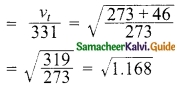= 1.080
vt = 331 × 1.080 = 357.48 m/s.
∴ Velocity of sound in air at that temperature = 357.48 m/s.

Question 4.
Explain why the ceilings of concert halls are curved.

1. These are basically curved surfaces (concave), which are used in auditoria and halls to improve the quality of sound. This board is placed such that the speaker is at the focus of the concave surface.
2. The sound of the speaker is reflected towards the audience thus improving the quality of sound heard by the audience.

Question 5.
Mention two cases in which there is no Doppler effect in sound?

1. When source (S) and listener (L) both are at rest.
2. When S and L move in such a way that distance between them remains constant.

VIII. Problem Corner:

Question 1.
A sound wave has a frequency of 200 Hz and a speed of 400 ms-1 in a medium. Find the wavelength of the sound wave.
Speed of sound wave v = 400 m/s
Frequency of sound wave n = 200 Hz
∴ Wavelength sound wave λ = $$\frac{v}{n}$$
= $$\frac{400}{200}$$ = 2 m
∴ Wavelength = 2 mQuestion 2.
The thunder of the cloud is heard 9.8 seconds later than the flash of lightning. If the speed of sound in air is 330 ms-1, what will be the height of the cloud?
Solution:
The height of the cloud (distance) = speed of sound × time
d = v × t = 330 × 9.8 = 3234 m
The height of the cloud is 3234 m.

Question 3.
A person who is sitting at a distance of 400 m from a source of sound is listening to a sound of 600 Hz. Find the time period between successive compressions from the source?
Distance of the observer = 400 m
Frequency of sound n = 600 Hz
Let velocity of sound be v = 330 $$\frac{m}{s}$$
Wavelength λ = $$\frac{v}{n}$$ = $$\frac{330}{600}$$
= 55 × 10-2 = 0.55 m
Distance between two successive compressions is $$\frac{λ}{2}$$
Time period successive compressions is = $$\frac{1}{\frac{λ}{2}}$$
= $$\frac{2}{λ}$$ = $$\frac{2}{0.55}$$
T = $$\frac{2}{0.55}$$ = 3.6363 second
Time period = 3.6363 second.

Question 4.
An ultrasonic wave is sent from a ship towards the bottom of the sea. It is found that the time interval between the transmission and reception of the wave is 1.6 seconds. What is the depth of the sea, if the velocity of sound in the seawater is 1400 ms-1?
Time interval t = 1.6 s
Velocity of sound in water = 1400 m/s.
Depth of the sea = $$\frac{v×t}{2}$$
= $$\frac{1400×1.6}{2}$$
= 1120 m
Depth of the sea = 1120 m

Question 5.
A man is standing between two vertical walls 680 m apart. He claps his hands and hears two distinct echoes after 0.9 seconds and 1.1 second respectively. What is the speed of sound in the air?
Distance between two walls λ = 680 m.
Time periods T1 = 0.9 s
T2 = 1.1 s
∴ Frequencies n1 = $$\frac{1}{T_1}$$ = $$\frac{1}{0.9}$$ = $$\frac{10}{9}$$
= 1.11 Hz
n2 = $$\frac{1}{T_2}$$
= $$\frac{1}{1.1}$$ = $$\frac{10}{11}$$
= 0.9090 Hz.
Difference x1 – x2 = 1.11 – 0.9090
= 0.201 Hz
Speed of sound = (n1 – n2
= 0.201 × 680 = 136.68

Question 6.
Two observers are stationed in two boats 4.5 km apart. A sound signal sent by one, underwater, reaches the other after 3 seconds. What is the speed of sound in the water?
Solution:
Distance between the two boats, d = 4.5 km
d = 4500 m
Time, t = 3 seconds
Speed of sound in the water = $$\frac{\text { Distance travelled by sound }}{\text { Time taken }}$$ = $$\frac{4500}{3}$$
v = 1500 ms-1.

Question 7.
A strong sound signal is sent from a ship towards the bottom of the sea. It is received back after Is.What is the depth of sea given that the speed of sound in water 1450 ms-1?
Speed of sound in water v = 1450 m/s
Time taken t = 1 + 1 = 2 s.
Depth of the sea d = $$\frac{v}{t}$$
= $$\frac{1450}{2}$$
= 725 m
Depth of the sea = 725 m.

Question 1.
What are the factors that affect the speed of sound in gases?
Effect of density:
The velocity of sound in a gas is inversely proportional to the square root of the density of the gas. Hence, the velocity decreases as the density of the gas increases.
$$v \propto \sqrt{\frac{1}{d}}$$

Effect of temperature:
(i) The velocity of sound in a gas is directly proportional to the square root of its temperature.
(ii) The velocity of sound in gas increases with the increase in temperature,
v ∝ √T Velocity at temperature T is given by the following equation:
vT = (v0 + 0.61 T) ms-1
Here, v0 is the velocity of sound in the gas at 0° C. For air, v0 = 331 ms-1.
Hence, the velocity of sound changes by 0.61 ms-1 when the temperature changes by one – degree celsius.

Effect of relative humidity: When humidity increases, the speed of sound increases. That is why we can hear sound from long distances clearly during rainy seasons.

Question 2.
What is mean by reflection of sound? Explain:
(a) Reflection at the boundary of a rarer medium.
(b) Reflection at the boundary of a denser medium.
(c) Reflection at curved surfaces.
When sound waves travel in a given medium and strike the surface of another medium, they can be bounced back into the first medium. This phenomenon is known as reflection.
(a) Reflection at the boundary of a rarer medium :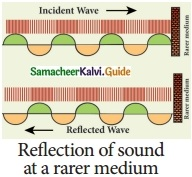Consider a wave travelling in a solid medium striking on the interface between the solid and the air. The compression exerts a force F on the surface of the rarer medium. As a rarer medium has smaller resistance for any defonnation, the surface of separation is pushed backwards. As the particles of the rarer medium are free to move, a rarefaction is produced at the interface. Thus, a compression is reflected as a rarefaction and a rarefaction travels from right to left.

(b) Reflection at the boundary of a denser medium :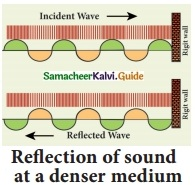A longitudinal wave travels in a medium in the form of compressions and rarefactions. Suppose a compression travelling in air from left to right reaches a rigid wall. The compression exerts a force F on the rigid wall. In turn, the wall exerts an equal and opposite reaction R = – F on the air molecules.

This results in a compression near the rigid wall. Thus, a compression travelling towards the rigid wall is reflected back as a compression. That is the direction of compression is reversed.

(c) Reflection at curved surfaces :
When the sound waves are reflected from the curved surfaces, the intensity of the reflected waves is changed. When reflected from a convex surface, the reflected waves are diverged out and the intensity is decreased. When sound is reflected from a concave surface, the reflected waves are converged and focused at a point. So the intensity of reflected waves is concentrated at a point.Question 3.
(a) What do you understand by the term ‘ultrasonic vibration’?
(b) State three uses of ultrasonic vibrations.
(c) Name three animals which can hear ultrasonic vibrations.
(a) Ultrasonic Vibration: The vibrations whose frequencies are greater than 20000 Hz are called ultrasonic vibrations.

(b) (i) They are used in SONAR to measure the depth of the sea (or ocean) and to locate underwater objects.
(ii) It is used for scanning and imaging the position and growth of a foetus and presence of stones in the gall bladder and kidney.
(iii) It is used for homogenising milk in milk plants where fresh milk is agitated with the desired quantity of fat and powdered milk to obtain toned milk.

(c) Mosquito, Dogs and Bats are the three animals that can hear ultrasonic vibrations.

Question 4.
What is an echo?
An echo is the sound reproduced due to the reflection of the original sound wave.

(a) State two conditions necessary for hearing an echo.
(b) What are the medical applications of echo?
(c) How can you calculate the speed of sound using echo?
(a) (i) The persistence of hearing for human ears is 0.1 second. This means that you can hear two sound waves clearly, if the time interval between the two sounds is at least 0.1 s. Thus, the minimum time gap between the original sound and an echo must be 0.1 s.

(ii) The above criterion can be satisfied only when the distance between the source of sound and the reflecting surface would satisfy the following equation: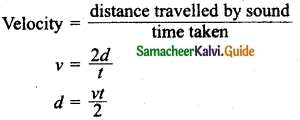Since, t = 0.1 second, then
d = $$\frac{v×0.1}{2}$$ = $$\frac{v}{20}$$
Thus the minimum distance required to hear an echo is 1/20th part of the magnitude of the velocity of sound in air. If you consider the velocity of sound as 344 ms-1, the minimum distance required to hear an echo is 17.2 m.

(b) The principle of echo is used in obstetric ultrasonography, which is used to create real-time visual images of the developing embryo or foetus in the mother’s uterus. This is a safe testing tool, as it does not use any harmful radiations.

(c) The sound pulse emitted by the source travels a total distance of 2d while travelling from the source to the wall and then back to the receiver. The time taken for this has been observed to be ‘t’. Hence, the speed of sound wave is given by:
Speed of sound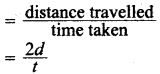X. HOT Questions

Question 1.
Suppose that a sound wave and a light wave have the same frequency, then which one has a longer wavelength?
(a) Sound, (b) Light, (c) both a and b, (d) data not sufficient
(b) Light: Since the velocity of light (C = 3 × 108 m/s) is greater than the velocity of sound, light wave has a longer wavelength.

Question 2.
When sound is reflected from a distant object, an echo is produced. Let the distance between the reflecting surface and the source of sound remain the same. Do you hear an echo sound on a hotter day? Justify your answer.
An echo can only be heard if it reaches the ear after 0.1 secs.
$$\text { Time taken }=\frac{\text { Totaldistance }}{\text { Velocity }}$$
As on a hotter day, the velocity of sound is more. So if the time taken by the echo is less than 0.1 sec than it won’t be heard.Question 1.
Sound waves are:
(a) Transverse waves
(b) Longitudinal waves
(c) Waves
(d) None of the above
(b) Longitudinal waves

Question 2.
The velocity of sound in air is ______.
(a) faster in dry air than in moist air
(b) directly proportional to temperature.
(c) directly proportional to pressure.
(d) Slower in dry air than in moist air.
(b) directly proportional to temperature.
Hint: v ∝ √T

Question 3.
Which of the following does not affect the velocity of sound?
(a) mass of the gas
(b) density of the gas
(c) temperature of the gas
(d) pressure of the gas
(d) pressure of the gas

Question 4.
The apparent frequency in Doppler’s effect does not depend upon.
(a) Speed of the listener.
(b) Distance between the listener and the source.
(c) Speed of the source.
(d) Frequency of the source.
(b) Distance between the listener and the source.
Hint: Apparent frequency in Doppler’s effect depends on frequency of the source, direction and velocity of source and listener. It does not depend on the distance between the listener and the source.

Question 5.
Sound of frequency 256 Hz passes through a medium. The maximum displacement is 0.1 m. The maximum velocity is:
(a) 60π m/s
(b) 30π m/s
(c) 51.2π m/s
(d) 512π m/s
(c) 51.2π m/sQuestion 6.
The speed of a wave in a medium is 760 ms-1. If 3600 waves the medium in 2 min, then its wavelength is ______.
(a) 13.8 m
(b) 41.5 m
(c) 25.3 m
(d) 57.2 m.
(c) 25.3 m
Hint: Number of waves crossing a point per second.
$$\begin{array}{l}{n=\frac{3600}{2 \times 60}=30} \\ {\lambda=\frac{v}{n}=\frac{760}{30}=25.3 \mathrm{m}}\end{array}$$.

Question 7.
Two waves are propagating with same amplitude and nearly same frequency in opposite they result in:
(a) longitudinal wave
(b) transverse wave
(c) stationary wave
(d) resonance wave
(c) stationary wave

Question 8.
Of these properties of a sound wave, the one that is independent of others is its:
(a) speed
(b) frequency
(c) wavelength
(d) amplitude
(d) amplitude

Question 9.
No echo will be heard if the distance between the reflecting obstacle and the source is:
(a) greater than 17.2 m
(b) less than 17.2 m
(c) less than 34.4 m
(d) greater than 34.4 m
(b) less than 17.2 m

Question 10.
The velocity of sound in a gas is independent of:
(a) temperature
(b) density
(c) humidity
(d) pressure
(d) pressure

Question 11.
The velocity of sound increases for every degree rise of temperature by:
(a) 1 ms-1
(b) 330 m/s
(c) 0.61 ms-1
(d) 6.1 m/s
(c) 0.61 ms-1

Question 12.
Longitudinal waves are characterised by:
(a) crest and troughs
(b) compressions and rarefactions
(c) nodes and antinodes
(d) wavelength and frequency
(b) compressions and rarefactions

Question 13.
If the frequency of waves lies between 20 Hz and 20 KHz then, they are:
(a) infrasonic waves
(b) ultrasonic waves
(c) audible waves
(d) transverse waves
(c) audible waves

Question 14.
The frequency of an infrasonic wave is:
(a) 20 Hz
(b) below 20 Hz
(c) above 20 Hz
(d) above 20 KHz
(b) below 20 Hz

Question 15.
The sound waves having frequency greater than 20 KHz are known as:
(a) audible waves
(b) ultrasonic waves
(c) infrasonic waves
(d) mechanical waves
(b) ultrasonic waves

Question 16.
Which of the following waves cannot be detected by human ear?
(a) audible wave
(b) infrasonic wave
(c) ultrasonic wave
(d) mechanical wave
(c) ultrasonic wave

Question 17.
Which waves are generated by stretched strings?
(a) audible waves
(b) sound waves
(c) ultrasonic waves
(d) infrasonic waves
(a) audible waves

Question 18.
The waves produced during earthquake, ocean waves are known as:
(a) audible waves
(b) infrasonic waves
(c) ultrasonic waves
(d) mechanical waves
(b) infrasonic waves

Question 19.
Sound wave belong to:
(a) mechanical waves
(b) electromagnetic waves
(c) transverse waves
(d) waves
(a) mechanical wavesQuestion 20.
The waves produced by bats are called:
(a) infrasonic waves
(b) ultrasonic waves
(c) electromagnetic waves
(d) mechanical waves
(b) ultrasonic waves

Question 21.
At N.T.P speed of sound waves in air is about:
(a) 3 x 108 m/s
(b) 3 x 10″8 m/s
(c) 340 cm/s
(d) 340 m/s
(d) 340 m/s

Question 22.
Sound waves are:
(a) mechanical waves
(b) longitudinal waves
(c) transverse waves
(d) waves
(b) longitudinal waves

Question 23.
The velocity of sound is least in ………. medium.
(a) solid
(b) liquid
(c) gaseous
(d) water
(c) gaseous

Question 24.
Velocity of sound ……… as the density of the solid increases.
(a) increases
(b) decreases
(c) changes
(d) none of the above
(b) decreases

Question 25.
Velocity of sound at a temperature T is given by:
(a) VT = (V0 + 0.61 T)
(b) VT = $$\frac{V_0}{273}$$
(c) VT = (V0 – 0.61 T)
(d) VT = V0(0.61T)
(a) VT = (V0 + 0.61 T)

Question 26.
In the case of reflection of sound waves angle of incidence is:
(a) less than angle of incidence
(b) equal to angle of incidence
(c) greater than angle of incidence
(d) greater than angle of refraction.
(b) equal to angle of incidence

Question 27.
The direction of compression is reversed during:
(a) refraction
(b) reflection at the boundary of a denser medium
(c) reflection at the boundary of a rarer medium
(d) reflection at the boundary of a curved surface
(b) reflection at the boundary of a denser medium

Question 28.
Which of the following property of sound waves is used in ultrasonography?
(a) Reflection of sound
(b) Refraction of sound
(c) Echo sound
(d) Doppler effect sound
(c) Echo sound

Question 29.
Which of the following is application of reflection of sound?
(a) Mega phone
(b) Ear trumpet
(c) Sound board
(d) All the above
(d) All the above

Question 30.
In Doppler effect when the source and listener move away from each other, the apparent frequency is given by: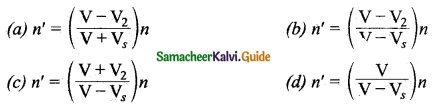(a)

Question 31.
When a listener approaches a stationary source, the apparent frequency is given by: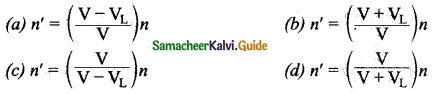(b)

Question 32.
If a source and a listener move towards each other, then the apparent frequency is: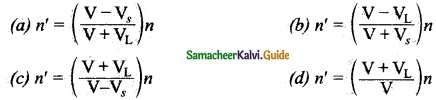(c)

Question 33.
When a source recedes from a stationary listener the apparent frequency is: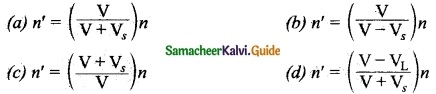(a)

Question 34.
When a source moves towards a stationary listener the ratio of apparent frequency to the original frequency is: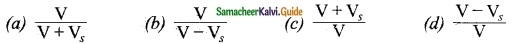(b)

Question 35.
When source and listener move one behind the other the apparent frequency is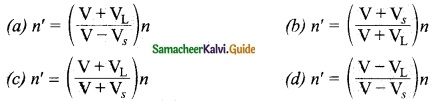(c)

II. Fill in the blanks:

1. The vibrating bodies produce waves in the form of ………
2. For the propagation of sound, a ………. is required.
3. The frequency range of audible waves is ………..
4. The frequency of infrasonic waves is …………
5. The sound waves with a frequency greater than 20 KHz are known as ………..
6. The wavelength of sound waves ranges from ………..
7. Sound waves are ……….
8. If wavelength of a wave is λ and its time period is T, then velocity of sound wave is ………..
9. If n is the frequency and λ is the wavelength of sound wave then is velocity of wave is given by …………
10. The ascending order of velocity of sound in solids, liquids and gases is given by …………
11. Velocity of sound in a gas is inversely proportional to the ………… of the medium.
12. Velocity of sound in solid is directly proportional to the square root of the ……….. of the medium.
13. Sound can be heard from long distances clearly during …………..
14. When the temperature of a gas changes by one degree Celsius, the velocity of sound changes by ………..
15. In the case of reflection of sound, angle of incidence is equal to ………….
16. When sound waves undergo reflection by convex surface, its intensity is ………..
17. ……….. are used when sound waves have to be focused at a point.
18. In ……….. surfaces, sound from one focus will always be reflected to the other focus.
19. The reproduction of sound due to the reflection of sound is known as …………
20. The minimum time gap between the original sound and an echo must be ………..
21. Echo is used to determine the velocity of ………… in any medium.
22. A horn-shaped device works on the principle of ………….
23. The phenomenon in which the frequency of sound heard by a listener is different from that of sound emitted by the source due to ……….. between a source and a listener is known as Doppler effect.
24. When the distance between the source and listener decreases, the apparent frequency is ………..
25. Apparent frequency in Doppler effect depends on the ……….. of the source and the listener.
26. When a listener moves away from a source at rest, the apparent frequency is n’ = ………..
27. When distance between source listener decreases, the apparent frequency is ………. than ……….. frequency.
28. If the medium moves with a velocity W in a direction opposite to the propagation of sound then, velocity of sound v becomes ………..
29. If the apparent frequency is n’ = ($$\frac{V}{V+V_{S}}$$)n then source moves ………….. the ……….. listener.
30. If a listener moves towards the ………. source, the apparent frequency n’ = ($$\frac{V+V_{L}}{V}$$)n.
1. sound waves
2. medium
3. From 20 Hz to 20 KHz
4. below 20 Hz
5. ultrasonic waves
6. 1.65 cm to 1.65 m
7. longitudinal
8. V = $$\frac{λ}{T}$$
9. V = nλ
10. VS > VL > VG
11. density
12. elastic modulus
13. rainy seasons
14. 0.61 m/s
15. the angle of reflection
16. decreased
17. parabolic surface
18. elliptical
19. echo
20. 0.1 second
21. sound waves
22. echo
23. relative motion
24. n’ = ($$\frac{V+V_{L}}{V-V_{S}}$$)n
25. velocities
26. ($$\frac{V+V_{L}}{V}$$)n
27. more, actual
29. [V – W]
29. away from, stationary
30. stationaryIII. True/False Questions: (If false give the reason)

1. Sound waves are transverse waves by nature.
2. Longitudinal waves are characterised by compressions and rarefactions.
3. Medium is not required for the propagation of light.
4. Ultrasonic waves are the waves with a frequency about 20 KHz.
5. Velocity of sound is the greatest in gas.
6. Velocity of sound in a gas is directly proportional to the square root of its temperature.
7. According to law of reflection of sound, angle of incidence is equal to angle of reflection.
8. When sound waves are reflected from a plane surface, the intensity of the reflected wave is neither decreased nor increased.
9. Ear trumpet works on the principle of reflection of sound.
10. The principle of SONAR is Doppler effect.
1. False – Sound waves are longitudinal waves by nature.
2. True
3. True
4. False – Ultrasonic waves are the waves with a frequency greater than 20 KHz.
5. False – Velocity of sound is the least in gas.
6. True
7. True
8. True
9. False – Ear trumpet works on the principle of reflection of sound.
10. True

IV. Match the following:

Question 1.
Match the Column I with Column II.(i) – (D)
(ii) – (E)
(iii) – (A)
(iv) – (B)

Question 2.
Match the Column I with Column II.(i) – (D)
(ii) – (A)
(iii) – (B)
(iv) – (E)

Question 3.
Match the Column I with Column II.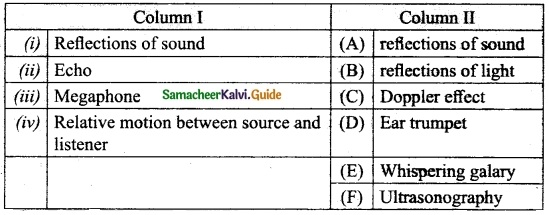(i) – (E)
(ii) – (F)
(iii) – (A)
(iv) – (C)

Question 4.
Match the Column I with Column II.(i) – (C)
(ii) – (E)
(iii) – (A)
(iv) – (D)

V. Assertion and Reason Questions:

Question 1.
Assertion: Sound waves cannot be propagated through vacuum but light can be transmitted.
Reason: Sound waves cannot be polarised but light wave can be polarised.
(a) If both the assertion and the reason are true and the reason is the correct explanation of the assertion.
(b) If both the assertion and the reason are true but the reason is not the correct explanation of the assertion.
(c) If the assertion is true, but the reason is false.
(d) If the assertion is false, but the reason is true.
(b) If both the assertion and the reason are true but the reason is not the correct explanation of the assertion.

Question 2.
Assertion: Ocean waves hitting a beach are always found to be nearly normal to the shore.
Reason: Ocean waves hitting a beach are assumed to be plane waves.
(a) If both the assertion and the reason are true and the reason is the correct explanation of the assertion.
(b) If both the assertion and the reason are true but the reason is not the correct explanation of the assertion.
(c) If the assertion is true, but the reason is false.
(d) If the assertion is false, but the reason is true.
(a) If both the assertion and the reason are true and the reason is the correct explanation of the assertion.

Question 3.
Assertion: Speed of a wave is the ratio between wavelength and time period of a wave.
Reason: Wavelength is the distance between two nearest particles in phase.
(a) If both the assertion and the reason are true and the reason is the correct explanation of the assertion.
(b) If both the assertion and the reason are true but the reason is not the correct explanation of the assertion.
(c) If the assertion is true, but the reason is false.
(d) If the assertion is false, but the reason is true.
(b) If both the assertion and the reason are true but the reason is not the correct explanation of the assertion.

Question 4.
Assertion: When a people moves along the sand within few centimetres of a sand scorpion, the scorpion immediately turns towards the people and dashes towards it.
Reason: When a people disturbs the sand, it sends pulses along the sand’s surface. One set of pulses is longitudinal and other set is transverse.
(a) If both the assertion and the reason are true and the reason is the correct explanation of the assertion.
(b) If both the assertion and the reason are true but the reason is not the correct explanation of the assertion.
(c) If the assertion is true, but the reason is false.
(d) If the assertion is false, but the reason is true.
(a) If both the assertion and the reason are true and the reason is the correct explanation of the assertion.Question 5.
Assertion: Light waves are transverse.
Reason: Light waves travel in air with a velocity of 3 × 108 m/s .
(a) If both the assertion and the reason are true and the reason is the correct explanation of the assertion.
(b) If both the assertion and the reason are true but the reason is not the correct explanation of the assertion.
(c) If the assertion is true, but the reason is false.
(d) If the assertion is false, but the reason is true.
(b) If both the assertion and the reason are true but the reason is not the correct explanation of the assertion.

Question 6.
Assertion: Velocity of sound in a gas is inversely proportional to the density of a gas.
Reason: When humidity increases the velocity of sound increases.
(a) If both the assertion and the reason are true and the reason is the correct explanation of the assertion.
(b) If both the assertion and the reason are true but the reason is not the correct explanation of the assertion.
(c) If the assertion is true, but the reason is false.
(d) If the assertion is false, but the reason is true.
(a) If both the assertion and the reason are true and the reason is the correct explanation of the assertion.

Question 7.
Assertion: Echo is produced due to the reflection of sound from walls, mountains etc.
Reason: Echo is used in ultrasonography.
(a) If both the assertion and the reason are true and the reason is the correct explanation of the assertion.
(b) If both the assertion and the reason are true but the reason is not the correct explanation of the assertion.
(c) If the assertion is true, but the reason is false.
(d) If the assertion is false, but the reason is true.
(b) If both the assertion and the reason are true but the reason is not the correct explanation of the assertion.

Question 8.
Assertion: Doppler effect is due to relative motion between the source and listener.
Reason: Echo is used in RADAR and SONAR.
(a) If both the assertion and the reason are true and the reason is the correct explanation of the assertion.
(b) If both the assertion and the reason are true but the reason is not the correct explanation of the assertion.
(c) If the assertion is true, but the reason is false.
(d) If the assertion is false, but the reason is true.
(d) If the assertion is false, but the reason is true.

Question 1.
What is the nature of sound waves?
Sound waves travel through any medium in the form of longitudinal waves.

Question 2.
How is the sound produced?
By touching a ringing bell or a musical instrument while it is producing music, we conclude that sound is produced by vibrations.

Question 3.
A wave travelling in a medium has time period T and wavelength λ. How T and λ are related?
Wave velocity v = $$\frac{λ}{T}$$
(or) λ = vT

Question 4.
Why don’t we hear sounds when our ears are closed?

• We hear the sound even when we close our ears but its magnitude and frequency is very less due to the obstruction of our hands.
• It also depends upon the frequency and loudness of the sound.

Question 5.
What is the relation between frequency and wavelength?
Wave velocity = Frequency × Wavelength
v = vλ

Question 6.
What do you understand by the term ‘infrasonic vibration’?
Infrasonic waves: These are sound waves with a frequency below 20 Hz that cannot be heard by the human ear. E.g., waves produced during an earthquake, ocean waves, the sound produced by whales, etc.

Question 7.
What is the range of audible frequency?
The audible range of frequency is from 20 Hz to 20 KHz.Question 1.
State the differences between sound and light.Question 2.
What is meant by particle velocity?
The velocity with which the particles of the medium vibrate in order to transfer the energy in the form of a wave is called particle velocity.

Question 3.
What do you know about wave velocity?
The velocity with which the wave travels through the medium is called wave velocity. In other words, the distance travelled by a sound wave in unit time is called the velocity of a sound wave.
∴ velocity = $$\frac{Distance}{Time taken}$$

Question 4.
Define wave velocity.
Wave velocity is defined as the distance travelled per second by a sound wave. Since,
Frequency (n) = 1/T, V = $$\frac{λ}{T}$$ can be written as V = nλ.

Question 5.
What is the effect of density of a gas on the velocity of sound?
The velocity of sound in a gas is inversely proportional to the square root of the density of the gas. Hence, the velocity decreases as the density of the gas increases.
V ∝$$\sqrt{\frac{1}{d}}$$

Question 6.
What is the effect of temperature of a gas on the velocity of sound?
The velocity of sound in a gas is directly proportional to the square root of its temperature. The velocity of sound in a gas increases with the increase in temperature.
V ∝ √T . Velocity at temperature T is given by the following equation:
VT = (V0 + 0.61T) ms2

Question 7.
What are the factors affect the speed of sound in gases?

• Effect of density
• Effect of temperature
• Effect of humidity

Question 8.
What do you know about reflection of sound from a plane surface?
When sound waves are reflected from a plane surface, the reflected waves travel in a direction, according to the law of reflection. The intensity of the reflected wave is neither decreased nor increased.

Question 9.
Write a note on whispering gallery.
One of the famous whispering galleries is in St. Paul’s cathedral church in London. It is built with elliptically shaped walls. When a person is talking at one focus, his voice can be heard distinctly at the other focus. It is due to the multiple reflections of sound waves from the curved walls.

Question 10.
How is echo of sound produced?
An echo is the sound reproduced due to the reflection of the original sound from various rigid surfaces such as walls, ceilings, surfaces of mountains, etc…

Question 11.
Write short notes about mega phone.
A megaphone is a horn-shaped device used to address a small gathering of people. Its one end is wide and the other end is narrow. When a person speaks . at the narrow end, the sound of his speech is concentrated by the multiple reflections from the walls of the tube. Thus, his voice can be heard loudly over a long distance.Question 12.
Define Doppler effect.
When ever there is a relative motion between a source and a listener, the frequency of the sound heard by the listener is different from the original frequency of sound emitted by the source. This is known as “Doppler effect”.

Question 13.
How Doppler effect is used to measure the speed of an automobile?
An electromagnetic wave is emitted by a source attached to a police car. The wave is reflected by a moving vehicle, which acts as a moving source. There is a shift in the frequency of the reflected wave. From the frequency shift, the speed of the car can be determined. This helps to track the over speeding vehicles.

Question 14.
How is Doppler effect utilised in tracking a satellite?
The frequency of radio waves emitted by a satellite decreases as the satellite passes away from the Earth. By measuring the change in the frequency of the radio waves, the location of the satellites is studied.

Question 15.
How is the location of aircrafts found out?
In RADAR, radio waves are sent, and the reflected waves are detected by the receiver of the RADAR station. From the frequency change, the speed and location of the aeroplanes and aircrafts are tracked.

Question 16.
How is the speed of submarine estimated?
In SONAR, by measuring the change in the frequency between the sent signal and received signal, the speed of marine animals and submarines can be determined.

VIII. Numerical problems:

Question 1.
How far does the sound travel in air when a tuning fork of frequency 256 Hz makes 64 vibrations? Velocity of sound in air = 340 m/s².
Velocity of sound in air v = 340 m/s
Frequency v = 256 Hz
Distance travelled by the wave in one vibration is equal to its wavelength. Wavelength λ = $$\frac{v}{V}$$
= $$\frac{340}{256}$$ = 1.328 m
Distance travelled by the wave in 64 vibrations
= 1.328 × 64
= 84.992 m = 85 m

Question 2.
A train moving with velocity 30 ms-1 blows a whistle of frequency 516 Hz. Find the pitch of the sound heard by a passenger ¡n another train approaching the first at a speed of 15 ms-1, the velocity of sound = 330 ms-1.
Solution:
It both the trains are approaching each other.
The apparent frequency, $$n^{\prime}=\left(\frac{v+v_{o}}{v-v_{\mathrm{s}}}\right) n$$
$$\begin{array}{l}{n^{\prime}=\left(\frac{330+15}{330-30}\right) \times 516} \\ {n=\frac{345}{300} \times 516=1.15 \times 516} \\ {n^{\prime}=593.4 \mathrm{Hz}}\end{array}$$.

Question 3.
A body generates waves of 100 mm long through medium A and 0.25 m long in medium B. If the velocity of waves in medium A is 80 cm s-1. Then, calculate the velocity of waves in medium B.
In medium A wavelength λA = 100 mm
= 0.1 m
In medium A velocity vA = 80 m/s
= 0.8 m/s
In medium B let velocity be vB
In medium B, wavelength λB = 0.25 m
As the frequency of the wave remains same in the two media, so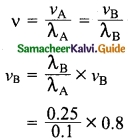= 2 m/s
∴ Velocity of waves in the medium B = 2 m/s

Question 4.
A radio station broadcasts its programme at 219.3 m wavelength. Determine the frequency of radio waves if velocity of radio waves 3 × 108 m/s.
Velocity of radio waves v = 3 × 108 m/s
Wavelength of radio waves λ = 219.3 m
∴ Frequency of radio waves v = $$\frac{v}{λ}$$
v = $$\frac{3×10^8}{219.3}$$
= 0.013679 × 108
= 13679 × 10² Hz
= 1.36 79 × 10³ Hz
Frequency v = 1.3679 KHz

Question 5.
The speed of a wave in a medium is 960 m/s. If 3600 waves are passing through a point in the medium in 1 minute, then calculate its wavelength.
Speed of a wave v = 960 m/s
Frequency of the wave v = 3600 m-1
$$\frac{3600}{60}$$ = 60 s-1
Wavelength λ = $$\frac{v}{V}$$
$$\frac{960}{60}$$ = 16 m
Wavelength = 16 mQuestion 6.
If a splash is heard 4.23 second after a stone is dropped into a well 78.4 m deep then calculate the speed of sound in air.
For downward motion of the stone,
Initial velocity u = 0
Acceleration a = 9.8 m/s²
Distance S = 74.8 m.
Time t = ?
S = ut + $$\frac{1}{2}$$ at²
78.4 = 0 + $$\frac{1}{2}$$ × 9.8 t²
= 4.9 t²
∴ t² = $$\frac{78.4}{4.9}$$ = 16
∴ t = 4 s
Let ‘t’ be the time taken by the splash of sound to reach the top of the well.
Then,
t + t’ = 4 +t’ = 4.23 s
∴ t = 4.23 – 4 = 0.23 s
∴ Speed of sound in air
$$\frac{distence}{Time}$$
= $$\frac{78.4}{0.23}$$
= 340.87 m/s

Question 7.
At what temperature will the speed of sound be double its value at 273 K?
We know that velocity of sound in a gas is inversely proportional to square root of its temperature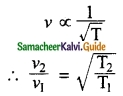T1 = 0°C = 0 + 273 = 273 K
v1 = 2v1 T2 = ?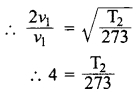∴ T2 = 4 × 273 = 1092 K
Required temperature = 1092 K

Question 8.
A listener moves towards a stationary source of sound with a velocity one fifth of the velocity of sound. What is the percentage increase in the apparent frequency?
Hence listener moves towards the source.
VL = –$$\frac{V}{5}$$, VS = 0
VL – Velocity of listener
VS – Velocity of source
V – Velocity of sound
Apparent frequency = n’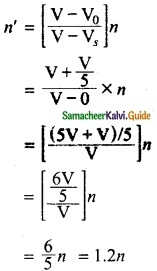The percentage increase in apparent frequency= 20%

Question 9.
A siren is fitted on a car going towards a vertical wall at a speed of 36 Kmph. A person standing on the ground behind the car, listens to the siren sound coming directly from the source as well as that coming after reflection from the wall. Calculate the apparent frequency of the wave coming directly from the siren to the person.
Velocity of source = 36 Kmph
VS = 36 × $$\frac{5}{18}$$ = 10 m/s
Velocity of sound v = 340 m/s
Frequency n = 500 Hz
For the sound coming directly from the siren to the listener, source (siren) is moving away from the listener so
VS = -10 m/s V2 = 0
Apparent frequency of the wave= 485.7 Hz

Question 10.
The sirens of two fire engines have a frequency of 600 Hz each. A man hears the sirens from the two engines, one approaching him with a speed of 30 Kmph and the car going away from him at a speed of 54 Kmph. What is the difference in frequency of two sirens heard by the man?
Velocity of sound be 340 m/s
Frequency n = 600 Hz
Velocity of sound
V = 340 m/s
Velocity of source
Vs = 36 Kmph
$$\frac{36×5}{18}$$ = 10 m/s
Velocity listener
VS = 0
For the engine approaching man= 618.2 Hz
For the engine going away from the man
VS = -54 Kmph
= -54 × $$\frac{5}{18}$$ = -15 m/s
Velocity of listener VL = 0
Apparent frequency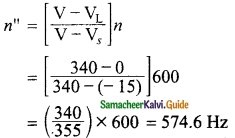Difference in frequencies = n’ – n” = 618.2 – 574.6
= 43.6 Hz

Question 1.
Explain the nature of sound waves.
Sound waves are longitudinal waves that can travel through any medium (solids, liquids, gases) with a speed that depends on the properties of the medium. As sound travels through a medium, the particles of the medium vibrate along the direction of propagation of the wave. This displacement involves the longitudinal displacements of the individual molecules from their mean positions. This results in a series of high and low pressure regions called compressions and rarefactions.Question 2.
Explain in the details of measuring the velocity of sound by the Echo method.
Apparatus required:
A source of sound pulses, a measuring tape, a sound receiver, and a stopwatch.
Procedure:

• Measure the distance d between the source of sound pulse and the reflecting surface using the measuring tape.
• The receiver is also placed adjacent to the source. A sound pulse is emitted by the source.
• The stopwatch is used to note the time interval between the instant at which the sound pulse is sent and the instant at which the echo is received by the receiver. Note the time interval as ‘t’.
• Repeat the experiment for three or four times. The average time taken for the given number of pulses is calculated.

Calculation of speed of sound:

• The sound pulse emitted by the source travels a total distance of 2d while travelling from the source to the wall and then back to the receiver.
• The time taken for this has been observed to be ‘t’. Hence, the speed of the sound wave is given by:
$$\text { Speed of sound }=\frac{\text { Distance travelled }}{\text { Time taken }}=\frac{2 d}{t}$$Question 3.
Explain laws of reflection with the help of a diagram.
The following two laws of reflection are applicable to sound waves as well.
(i) The incident wave, the normal to the reflecting surface and the reflected wave at the point of incidence lie in the same plane.
(ii) The angle of incidence ∠i is equal to the angle of reflection ∠r.
These laws can be observed from figure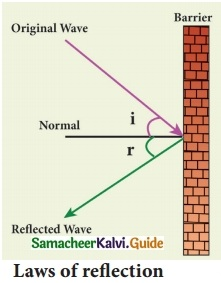In the above Figure, the sound waves that travel towards the reflecting surface are called the incident waves. The sound waves bouncing back from the reflecting surface are called reflected waves. For all practical purposes, the point of incidence and the point of reflection is the same point on the reflecting surface.

A perpendicular line drawn at the point of incidence is called the normal. The angle which the incident sound wave makes with the normal is called the angle of incidence, ‘i’. The angle which the reflected wave makes with the normal is called the angle of reflection, ‘r’.

Question 4.
Explain applications of echo.
(i) Some animals communicate with each other over long distances and also locate objects by sending the sound signals and receiving the echo as reflected from the targets.

(ii) The principle of echo is used in obstetric ultrasonography, which is used to create real-time visual images of the developing embryo or fetus in the mother’s uterus. This is a safe testing tool, as it does not use any harmful radiations.

(iii) Echo is used to determine the velocity of sound waves in any medium.

Question 5.
Explain various applications of Doppler effect.
1. To measure the speed of an automobile:
An electromagnetic wave is emitted by a source attached to a police car. The wave is reflected by a moving vehicle, which acts as a moving source. There is a shift in the frequency of the reflected wave. From the frequency shift, the speed of the car can be determined. This helps to track the over speeding vehicles.

2. Tracking a satellite:
The frequency of radio waves emitted by a satellite decreases as the satellite passes away from the Earth. By measuring the change in the frequency of the radio waves, the location of the satellites is studied.

In RADAR, radio waves are sent, and the reflected waves are detected by the receiver of the RADAR station. From the frequency change, the speed and location of the aeroplanes and aircraft are tracked.

4. SONAR:
In SONAR, by measuring the change in the frequency between the sent signal and received signal, the speed of marine animals and submarines can be determined.

Question 6.
Describe (i) Soundboard
(ii) Ear trumpet and
(iii) Megaphone.
(i) Sound Board: These are basically curved surfaces (concave), which are used in auditoria and halls to improve the quality of sound. This board is placed such that the speaker is at the focus of the concave surface. The sound of the speaker is reflected towards the audience thus improving the quality of sound heard by the audience.

(ii) Ear trumpet: Ear trumpet is a hearing aid, which is useful by people who have difficulty in hearing. In this device, one end is wide and the other end is narrow. The sound from the sources fall into the wide end and are reflected by its walls into the narrow part of the device. This helps in concentrating the sound and the sound enters the eardrum with more intensity. This enables a person to hear the sound better.

(iii) MegaPhone: A megaphone is a horn-shaped device used to address a small gathering of people. Its one end is wide and the other end is narrow. When a person speaks at the narrow end, the sound of his speech is concentrated by the multiple reflections from the walls of the tube. Thus, his voice can be heard loudly over a long distance.

Question 7.
What is the Doppler effect? Explain it in several situations.
Whenever there is a relative motion between a source and a listener, the frequency of the sound heard by the listener is different from the original frequency of sound emitted by the source. This is known as “Doppler effect”.

For simplicity of calculation, it is assumed that the medium is at rest, That is the velocity of the medium is zero. Let S and L be the source and the listener moving with velocities vS and vL respectively. Consider the case of source and listener moving towards each other. As the distance between them decreases, the apparent frequency will be more than the actual source frequency.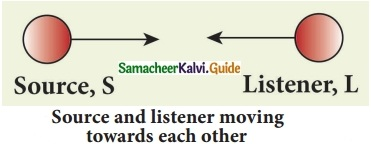Let n and n’ be the frequency of the sound produced by the source and the sound observed by the listener respectively. Then, the expression for the apparent frequency n’ is
n’ = ($$\frac{V+V_L}{V-V_S}$$)n
Here, v is the velocity of sound waves in the given medium. Let us consider different possibilities of motions of the source and the listener. In all such cases, the expression for the apparent frequency is given in tableSuppose the medium (say wind) is moving with a velocity W in the direction of the propagation of sound. For this case, the velocity of sound, ‘v’ should be replaced with (v + W). If the medium moves in a direction opposite to the propagation of sound, then ‘v’ should be replaced with (v – W).

Question 8.
Describe the applications of Doppler effect.
(i) To measure the speed of an automobile : An electromagnetic wave is emitted by a source attached to a police car. The wave is reflected by a moving vehicle, which acts as a moving source. There is a shift in the frequency of the reflected wave. From the frequency shift, the speed of the car can be determined. This helps to track the over speeding vehicles.

(ii) Tracking a satellite : The frequency of radio waves emitted by a satellite decreases as the satellite passes away from the Earth. By measuring the change in the frequency of the radio waves, the location of the satellites is studied.

(iii) RADAR (RAdio Detection And Ranging) : In RADAR, radio waves are sent, and the reflected waves are detected by the receiver of the RADAR station. From the frequency change, the speed and location of the aeroplanes and aircrafts are tracked.

(iv) SONAR (SOund Navigation And Ranging) : In SONAR, by measuring the change in the frequency between the sent signal and received signal, the speed of marine animals and submarines can be determined.Question 9.
Explain the conditions for no Doppler effect.
Under the following circumstances, there will be no Doppler effect and the apparent frequency as heard by the listener will be the same as the source frequency.

1. When source (S) and listener (L) both are at rest.
2. When S and L move in such a way that distance between them remains constant.
3. When source S and L are moving in mutually perpendicular directions.
4. If the source is situated at the center of the circle along which the listener is moving.

IX. Hot questions:

Question 1.
Why is frequency the most fundamental property of a wave?
When a waves travels from one medium to another, its velocity and wavelength may change. But the frequency does not change hence the frequency is the most fundamental property of a wave.

Question 2.
Which properties of solid are required for the propagation of sound?
Elasticity and inertia are the properties of solid are required for the propagation of sound.

Question 3.
What does cause the rolling sound of thunder?
The multiple reflections of sound of lightning produce the rolling sound of thunder.

Question 4.
On the surface of moon, two astronauts can not talk to each other. Give reason.
Sound waves require material medium for their propagation. As there is no ‘ atmosphere, on the moon, hence the sound wave can not propagate on the moon.

Question 5.
If a person places his ear to one end of a long iron pipeline, he can distinctly hear two sound waves when a workman hammers the other end of the pipeline. How?
Sound waves travel sixteen times faster in iron than in air. Hence the person hears two sounds, the first one travelling through the iron pipeline and the second travelling through air.

Question 6.
Sound waves travel faster in solids than in liquids and gases. Why?
Sound waves travel faster in solids with greatest speed. Because the coefficient of elasticity of solids is much greater than coefficient of elasticity of liquids and gases.Question 7.
If an explosion takes place at the bottom of a lake, will the shock waves in water be longitudinal or transverse?